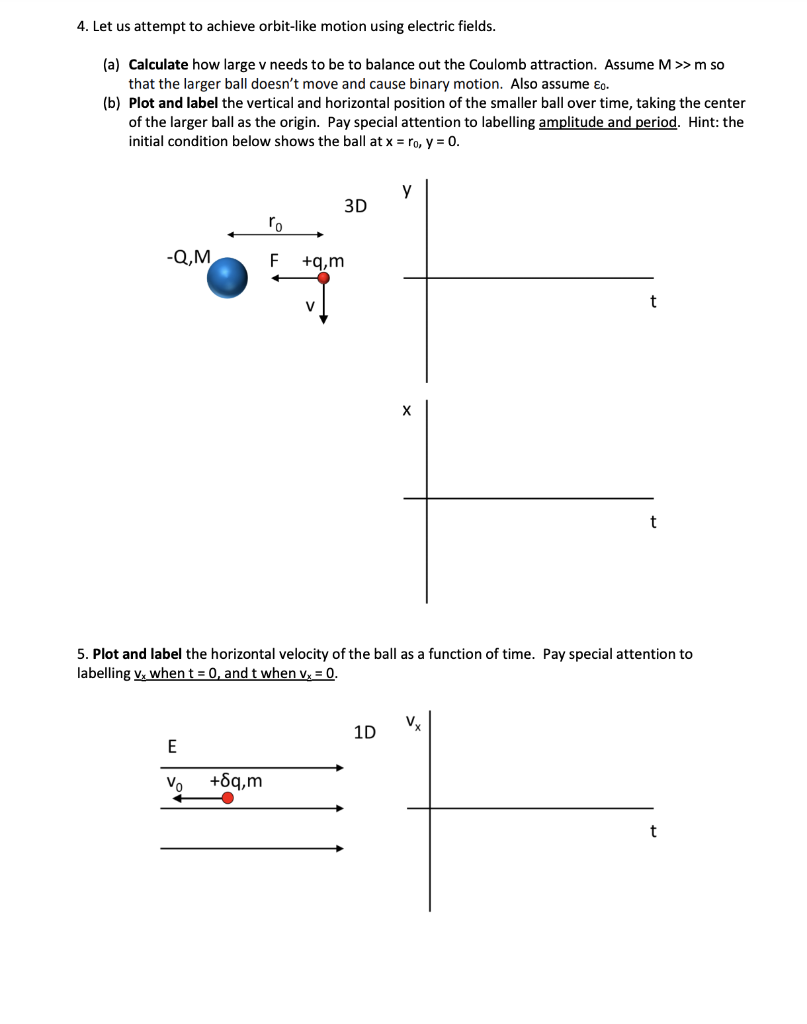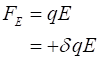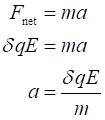# 4. Let us attempt to achieve orbit-like motion using electric fields.(a) Calculate how large v needs to be to balance out the Coulomb attraction. Assume M>>m sothat the larger ball doesn't move and cause binary motion. Also assume ɛg.(b) Plot and label the vertical and horizontal position of the smaller ball over time, taking the centerof the larger ball as the origin. Pay special attention to labelling amplitude and period. Hint: theinitial condition below shows the ball at x = ro, y = 0.3Dro-Q,M+g.mх5. Plot and label the horizontal velocity of the ball as a function of time. Pay special attention tolabelling v, when t = 0, and t when v, = 0.1DVo+6q,m

Question
1 viewshelp_outlineImage Transcriptionclose4. Let us attempt to achieve orbit-like motion using electric fields. (a) Calculate how large v needs to be to balance out the Coulomb attraction. Assume M>>m so that the larger ball doesn't move and cause binary motion. Also assume ɛg. (b) Plot and label the vertical and horizontal position of the smaller ball over time, taking the center of the larger ball as the origin. Pay special attention to labelling amplitude and period. Hint: the initial condition below shows the ball at x = ro, y = 0. 3D ro -Q,M +g.m х 5. Plot and label the horizontal velocity of the ball as a function of time. Pay special attention to labelling v, when t = 0, and t when v, = 0. 1D Vo +6q,m fullscreen
check_circle

Step 1

Since you have asked multiple question, we will solve the question number 6 for you. If you
want any specific question to be solved then please specify the question number or post
only that question.

The formula for the electrostatic force acting on a charged particle is:The only force acting on the charged particle along the x-direction is electrostatic force. Therefore, from Newton’s second law of motion.Step 2

In the expression of acceleration, all the terms are constant. Therefore, the acceleration acting on the charged particle will be constant. Initially, the direction of acceleration is opposite to the direction of velocity. Therefore, the particle velocity will decrease until the velocity reaches zero, after that the velocity reverses its dire...

### Want to see the full answer?

See Solution

#### Want to see this answer and more?

Solutions are written by subject experts who are available 24/7. Questions are typically answered within 1 hour.*

See Solution
*Response times may vary by subject and question.
Tagged in